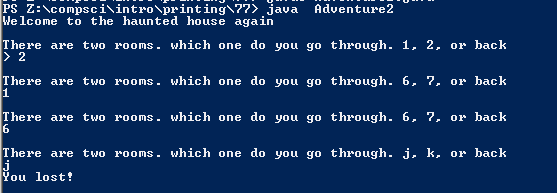PROGRAMMING AND CODE

# Assignemnt #77

## Code

```/// Name: Justin Li
/// Period: 7
/// Date Completed: 2/11/16

import java.util.Scanner;

public static void main(String[] args) {

Scanner keyboard = new Scanner(System.in);

int n = 0;
String a, b, c;
a = "";

System.out.println("Welcome to the haunted house again");

while (n == 0) {
if (a.equals("")) {
System.out.println("\nThere are two rooms. which one do you go through. 1, 2, or back");

System.out.print("> ");
a = keyboard.next();
}

if (a.equals("1")) {
System.out.println("There are two rooms. which one do you go through. 3, 4, or back.");
b = keyboard.next();

if (b.equals("3")) {
System.out.println("There are two rooms. which one do you go through. a, b, or back.");
c = keyboard.next();

if (c.equals("a")) {
System.out.println("Sorry you lost!");

}
else if (c.equals("b")) {
System.out.println("Sorry you lost!");

}
else if (c.equals("back"))
b = "N/A";
}
else if (b.equals("4")) {
System.out.println("There are two rooms. which one do you go through. x, y, or back.");
c = keyboard.next();

if (c.equals("x")) {
System.out.println("Sorry you lost!");
}
else if (c.equals("y")) {
System.out.println("Sorry you lost!");

}
else if (c.equals("back"))
c = "N/A";
}
else if (b.equals("back"))
a = "";
}
else if (a.equals("2")) {
System.out.println("\nThere are two rooms. which one do you go through. 6, 7, or back");
b = keyboard.next();

if (b.equals("6")) {
System.out.println("\nThere are two rooms. which one do you go through. j, k, or back");
c = keyboard.next();

if (c.equals("j")) {
System.out.println("You lost!");

}
else if (c.equals("k")) {
System.out.println("You have Won!");

}
else if (c.equals("back"))
c = "N/A";
}
else if (b.equals("7")) {
System.out.println("\nThere are two rooms. which one do you go through. 1, 0, or back");
c = keyboard.next();

if (c.equals("1")) {
System.out.println("You have won!");

}
else if (c.equals("0")) {
System.out.println("You have died.");
}
else if (c.equals("back"))
c = "N/A";
else if (b.equals("back"))
b = "N/A";
}
else if (b.equals("back"))
a = "";
}
}

System.out.println("\n\nThe end.");
}
}
```

### Picture of the output